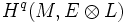# Kodaira vanishing theorem

This is a vanishing theorem

## Statement

Let$L$ be a holomorphic line bundle on a compact Kahler manifold$M$, whose topological Chern class can be represented by a closed$(1,1)$-form which is positive definite. Let$E$ be any other holomorphic vector bundle. Then the cohomology groups$H^q(M, E \otimes L)$ vanish for large enough$r$ whenever$q$ is at least 1.

## Relation with other results

### Kodaira embedding theorem

Further information: Kodaira embedding theorem

### Lichnerowicz vanishing theorem

Further information: Lichnerowicz vanishing theorem